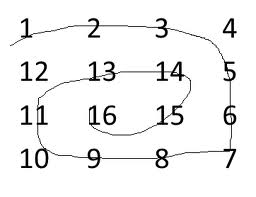# Java Program to Print matrix in antispiral form

Given a 2D array, the task is to print matrix in anti spiral form:
Examples:Output: 16 15 14 13 12 11 10 9 8 7 6 5 4 3 2 1

```Input : arr[] = {1, 2, 3, 4
5, 6, 7, 8
9, 10, 11, 12
13, 14, 15, 16};
Output : 10 11 7 6 5 9 13 14 15 16 12 8 4 3 2 1

Input :arr[] = {1, 2, 3, 4, 5, 6
7, 8, 9, 10, 11, 12
13, 14, 15, 16, 17, 18};
Output : 11 10 9 8 7 13 14 15 16 17 18 12 6 5 4 3 2 1```

The idea is simple, we traverse matrix in spiral form and put all traversed elements in a stack. Finally one by one elements from stack and print them.

## Java

 `// Java Code for Print matrix in antispiral form` `import` `java.util.*;`   `class` `GFG {` `    `  `    ``public` `static` `void` `antiSpiralTraversal(``int` `m, ``int` `n, ` `                                             ``int` `a[][])` `    ``{` `        ``int` `i, k = ``0``, l = ``0``;` `     `  `        ``/*  k - starting row index` `            ``m - ending row index` `            ``l - starting column index` `            ``n - ending column index` `            ``i - iterator  */` `        ``Stack stk=``new` `Stack();` `     `  `        ``while` `(k <= m && l <= n)` `        ``{` `            ``/* Print the first row from the remaining ` `             ``rows */` `            ``for` `(i = l; i <= n; ++i)` `                ``stk.push(a[k][i]);` `            ``k++;` `     `  `            ``/* Print the last column from the remaining` `            ``columns */` `            ``for` `(i = k; i <= m; ++i)` `                ``stk.push(a[i][n]);` `            ``n--;` `     `  `            ``/* Print the last row from the remaining ` `            ``rows */` `            ``if` `( k <= m)` `            ``{` `                ``for` `(i = n; i >= l; --i)` `                    ``stk.push(a[m][i]);` `                ``m--;` `            ``}` `     `  `            ``/* Print the first column from the remaining ` `            ``columns */` `            ``if` `(l <= n)` `            ``{` `                ``for` `(i = m; i >= k; --i)` `                    ``stk.push(a[i][l]);` `                ``l++;` `            ``}` `        ``}` `     `  `        ``while` `(!stk.empty())` `        ``{` `            ``System.out.print(stk.peek() + ``" "``);` `            ``stk.pop();` `        ``}` `    ``}` `    `  `    ``/* Driver program to test above function */` `    ``public` `static` `void` `main(String[] args) ` `    ``{` `         ``int` `mat[][] =` `                ``{` `                    ``{``1``,  ``2``,  ``3``,  ``4``,  ``5``},` `                    ``{``6``,  ``7``,  ``8``,  ``9``,  ``10``},` `                    ``{``11``, ``12``, ``13``, ``14``, ``15``},` `                    ``{``16``, ``17``, ``18``, ``19``, ``20``}` `                ``};` `             `  `        ``antiSpiralTraversal(mat.length - ``1``, mat[``0``].length - ``1``, ` `                                                       ``mat);` `    ``}` `  ``}` `// This code is contributed by Arnav Kr. Mandal.`

Output:

`12 13 14 9 8 7 6 11 16 17 18 19 20 15 10 5 4 3 2 1 `

Time Complexity: O(m x n) where m is the number of rows and n is the number of columns in the matrix.

Space Complexity: O(m x n) as we are using a stack to store the elements.

Please refer complete article on Print matrix in antispiral form for more details!

Feeling lost in the world of random DSA topics, wasting time without progress? It's time for a change! Join our DSA course, where we'll guide you on an exciting journey to master DSA efficiently and on schedule.
Ready to dive in? Explore our Free Demo Content and join our DSA course, trusted by over 100,000 geeks!

Previous
Next